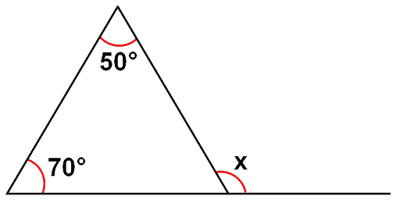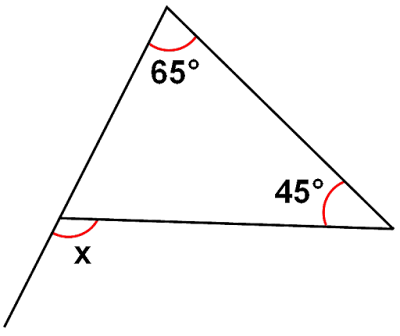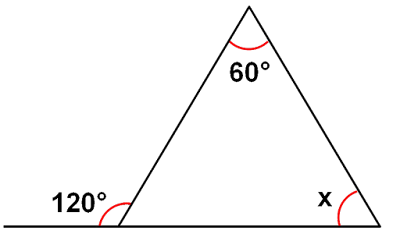# vs.eyeandcontacts.com

## Chapter 6 Triangle and its Properties Exercise 6.2

Question 1: Find the value of the unknown exterior angle x in the following diagram:
(i)(by using sum of 2 interior opposite angle is equal to the exterior angle)
= 50° + 70° = x
= 120° = x
Therefore, exterior angle x is equal to 120°.

(ii)(by using sum of 2 interior opposite angle is equal to the exterior angle)
= 65° + 45° = x
= 110° = x
Therefore, exterior angle x is equal to 110°.

(iii)(by using sum of 2 interior opposite angle is equal to the exterior angle)
= 30° + 40° = x
= 70° = x
Therefore, exterior angle x is equal to 70°.

(iv)(by using sum of 2 interior opposite angle is equal to the exterior angle)
= 60° + 60° = x
= 120° = x
Therefore, exterior angle x is equal to 120°.

(v)(by using sum of 2 interior opposite angle is equal to the exterior angle)
= 50° + 50° = x
= 100° = x
Therefore, exterior angle x is equal to 100°.

(vi)(by using sum of 2 interior opposite angle is equal to the exterior angle)
= 30° + 60° = x
= 90° = x
Therefore, exterior angle x is equal to 90°.

Question 2: Find the value of the unknown interior angle x in the following figures:
(i)(by using sum of 2 interior opposite angle is equal to the exterior angle)
= 50° + x = 115°
= 115° - 50° = 65°
= x = 65°
Therefore, interior angle x is equal to 65°.

(ii)(by using sum of 2 interior opposite angle is equal to the exterior angle)
= 70° + x = 100°
= 100° - 70° = 30°
= x = 30°
Therefore, interior angle x is equal to 30°.

(iii)(by using sum of 2 interior opposite angle is equal to the exterior angle)
= 90° + x = 125°
= 125° - 90° = 35°
= x = 35°
Therefore, interior angle x is equal to 35°.

(iv)(by using sum of 2 interior opposite angle is equal to the exterior angle)
= 60° + x = 120°
= 120° - 60° = 60°
= x = 60°
Therefore, interior angle x is equal to 60°.

(v)(by using sum of 2 interior opposite angle is equal to the exterior angle)
= 30° + x = 80°
= 80° - 30° = 50°
= x = 50°
Therefore, interior angle x is equal to 50°.

(vi)